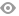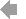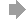﻿ Page 5 - MATINF Nr. 6
Basic HTML VersionView Full Version
Page 5 - MATINF Nr. 6
P. 5

``````˘
ARTICOLE SI NOTE DE MATEMATICA
,

Two Identities and their Consequences

Emmanuel Antonio Jos´e Garc´ıa          1

The Heron’s formula [1, 4, 7, 14, 15, 17, 18], named after Hero of Alexandria, gives the area of a
triangle when the length of all three sides are known. Indian mathematician and astronomer
Brahmagupta, in the seventh century, gave the analogous formula for a cyclic convex quadrilateral
[6, 10]. In 1842 German mathematician Carl Bretschneider related the area of a general convex
quadrilateral to its side lengths and the sum of two opposite angles [5, 8, 11]. Heron’s formula is
a special case of Brahmagupta’s formula for the area of a cyclic quadrilateral. Heron’s formula
and Brahmagupta’s formula are both special cases of Bretschneider’s formula for the area of a
In this note we prove the Heron’s formula (although known, see Conway’s dicussion in ),
the Brahmagupta’s formula (also known, see ) and the formula for the area of a bicentric
√
quadrilateral (possibly new, see [12, 13]),    abcd, based on two lesser-known trigonometric
formulae [6, 16] involving sine, cosine, the semiperimeter and the side lenghts of a cyclic
quadrilateral. Once the two trigonometric formulae have been established (and the necessary
adjustments made), the proofs of these area theorems are greatly simpliﬁed. Furthermore, we
present a generalization of the two aforementioned trigonometric formulae and use it to give
an alternative proof of Bretschneider’s formula. Since all these area theorems can be derived
from this new generalization, the approach presented in this note, unlike others, provides a
more holistic view of these theorems. Our main result for a general convex quadrilateral are the
identities
α           γ
ad sin 2  + bc cos 2  = (s − a)(s − d)
2           2
and
γ           α
bc sin 2  + ad cos 2  = (s − b)(s − c),
2            2

where a, b, c, d are the sides lengths, s is the semiperimeter, and α and γ are opposite angles.
We recall a cyclic quadrilateral is a quadrilateral whose vertices all lie on a single circle.
Among other characterizations, a convex quadrilateral ABCD is cyclic if and only if its opposite
◦
angles are supplementary, that is α + γ = 180  (see Figure 1).

Theorem 1. Let ABCD be a cyclic convex quadrilateral with AB = a, BC = b, CD = c,
DA = d and s =    a+b+c+d . If ∠BAD = α, then
2
α    (s − a)(s − d)               α    (s − b)(s − c)
sin 2  =                  and    cos 2  =                .                (1)1   2   3   4   5   6   7   8   9   10